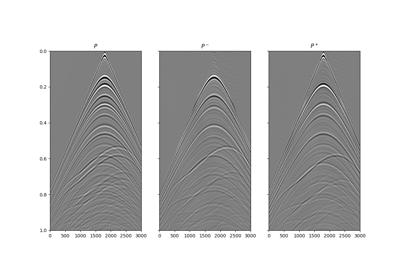# pylops.waveeqprocessing.Deghosting#

pylops.waveeqprocessing.Deghosting(p, nt, nr, dt, dr, vel, zrec, pd=None, win=None, npad=(11, 11), ntaper=(11, 11), restriction=None, sptransf=None, solver=<function lsqr>, dottest=False, dtype='complex128', **kwargs_solver)[source]#

Wavefield deghosting.

Apply seismic wavefield decomposition from single-component (pressure) data. This process is also generally referred to as model-based deghosting.

Parameters
pnp.ndarray

Pressure data of of size $$\lbrack n_{r_x}\,(\times n_{r_y}) \times n_t \rbrack$$ (or $$\lbrack n_{r_{x,\text{sub}}}\, (\times n_{r_{y,\text{sub}}}) \times n_t \rbrack$$ in case a restriction operator is provided. Note that $$n_{r_{x,\text{sub}}}$$ (and $$n_{r_{y,\text{sub}}}$$) must agree with the size of the output of this operator)

ntint

Number of samples along the time axis

nr

Number of samples along the receiver axis (or axes)

dtfloat

Sampling along the time axis

dr

Sampling along the receiver array of the separated pressure consituents

velfloat

Velocity along the receiver array (must be constant)

zrecfloat

pdnp.ndarray, optional

Direct arrival to be subtracted from p

winnp.ndarray, optional

Time window to be applied to p to remove the direct arrival (if pd=None)

ntaperfloat or tuple, optional

Number of samples of taper applied to propagator to avoid edge effects

npadfloat or tuple, optional

Number of samples of padding applied to propagator to avoid edge effects angle

restrictionpylops.LinearOperator, optional

Restriction operator

sptransfpylops.LinearOperator, optional

Sparsifying operator

solverfloat, optional

Function handle of solver to be used if kind='inverse'

dottestbool, optional

Apply dot-test

dtypestr, optional

Type of elements in input array. If None, directly inferred from p

**kwargs_solver

Arbitrary keyword arguments for chosen solver

Returns
pupnp.ndarray

Up-going wavefield

pdownnp.ndarray

Down-going wavefield

Notes

Up- and down-going components of seismic data $$p^-(x, t)$$ and $$p^+(x, t)$$ can be estimated from single-component data $$p(x, t)$$ using a ghost model.

The basic idea  is that of using a one-way propagator in the f-k domain (also referred to as ghost model) to predict the down-going field from the up-going one (excluded the direct arrival and its source ghost referred here to as $$p_d(x, t)$$):

$p^+ - p_d = e^{-j k_z 2 z_\text{rec}} p^-$

where $$k_z$$ is the vertical wavenumber and $$z_\text{rec}$$ is the depth of the array of receivers

In a matrix form we can thus write the total wavefield as:

$\mathbf{p} - \mathbf{p_d} = (\mathbf{I} + \Phi) \mathbf{p}^-$

where $$\Phi$$ is one-way propagator implemented via the pylops.waveeqprocessing.PhaseShift operator.

1

Amundsen, L., 1993, Wavenumber-based filtering of marine point-source data: GEOPHYSICS, 58, 1335–1348.

## Examples using pylops.waveeqprocessing.Deghosting#13. Deghosting

13. Deghosting